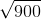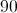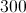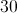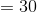# ISEE Middle Level Math : How to find the square root

## Example Questions

### Example Question #61 : Squares / Square Roots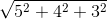The expression is equal to 7.

The expression is equal to 9.

The expression is equal to 8.

The expression falls between 8 and 9.

The expression falls between 7 and 8.

The expression falls between 7 and 8.

Explanation: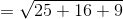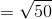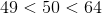, so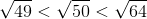, or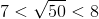### Example Question #62 : Squares / Square Roots

Simplify: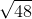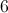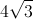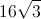Explanation: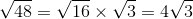### Example Question #63 : Squares / Square Roots

Let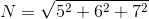.

Then which of the following statements is correct?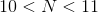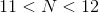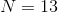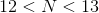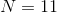Explanation: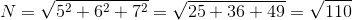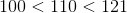, so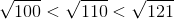### Example Question #64 : Squares / Square Roots

Let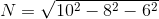.

Then which of the following statements is correct?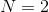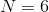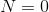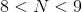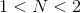Explanation: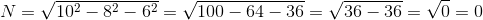### Example Question #65 : Squares / Square Roots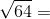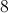Explanation:

Find the square root of 64: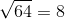### Example Question #66 : Squares / Square Roots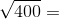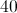Explanation:

Find the square root: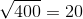### Example Question #67 : Squares / Square Roots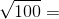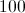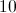Explanation:

Find the square root: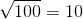### Example Question #68 : Squares / Square Roots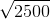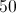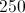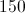Explanation:

Find the square root :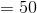### Example Question #69 : Squares / Square Roots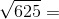Explanation:

Find the square root: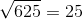### Example Question #70 : Squares / Square Roots